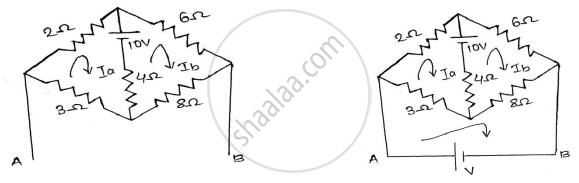# Using the Mesh Analysis Find the Mesh Current in the Direction Shown and Also Find the Voltage Across a and B Terminals . - Basic Electrical and Electronics Engineering

Sum

Using the mesh analysis find the mesh current in the direction shown and also find the voltage across A and B terminals.

#### Solution

Applying KVL to mesh a
−3𝐼𝑎 − 2𝐼𝑎 − 4(𝐼𝑎 − 𝐼𝑏 ) + 10 = 0
−3𝐼𝑎 − 2𝐼𝑎 − 4𝐼𝑎 + 4𝐼𝑏 + 10Applying KVL to mesh b

-10 + 6𝐼𝑎 + 8𝐼𝑏 − 4(𝐼𝑏 − 𝐼𝑎) = 0
6𝐼𝑏 + 8𝐼𝑏 − 4𝐼𝑏 + 4𝐼𝑎 = 10
-4𝐼𝑎 + 18𝐼𝑏 = 10 ………..(2)
From (1) and (2) we get,
𝐼𝑎 = 1.5068A and 𝐼𝑏 = 0.890A
For voltage:-
Apply KVL to mesh
-V+3𝐼𝑎 + 8𝐼𝑏 = 0
V = 3𝐼𝑎 + 8𝐼𝑏
V = 3× 1.5068 + 0.89 × 8
V = 11.640V

Concept: Mesh Analysis
Is there an error in this question or solution?